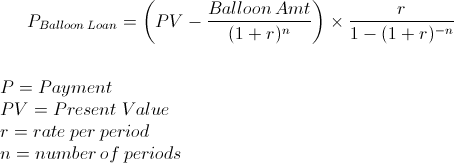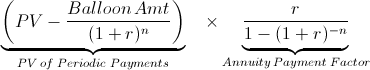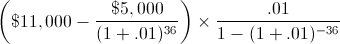Custom Search

# Payments on a Balloon Loan

Balloon Loan Payment Calculator (Click Here or Scroll Down)The balloon loan payment formula is used to calculate the payments on a loan that has a balance remaining after all periodic payments are made. Examples of loans that may use the balloon loan payment formula would be auto leases, balloon mortgages, and any other form of loan not paid in full at its end date.

The formula for a balloon loan payment could also be used for any form of annuity where a balance is left after all periodic cash flows are made. An annuity is simply a series of periodic payments. An example of how this formula could be applied in a non-loan related way would be if an individual has \$11,000 sitting in their interest account that must last them 2 years, and they need to have a balance of \$5,000 at the end of the 2nd year. The monthly amount withdrawn could be calculated using the balloon loan payment formula.

One may be enticed to calculate the example above by simply subtracting \$5,000 from \$11,000 and calculating the payment based on an ordinary annuity of \$6,000. However, one must consider that the \$5,000 will earn interest over the 2 years leaving a balance higher than \$5,000 after the 2nd year. This may be acceptable for a smaller amount or for quick calculations in ordinary life, however it is not the exact way to calculate the periodic payment.

### How is the Balloon Loan Payment Formula Derived?

The balloon loan payment formula can be found by first separating the two main parts of the formula.The 2nd section of the formula is simply the formula for the annuity payment factor which is used to calculate the payment on any type of annuity. Again, an annuity mentioned here is a series of periodic payments and not to be confused with the financial instrument. The payments or cash flows of an annuity can be found by multiplying the original balance or present value times the annuity payment factor.

The 1st portion of the formula is one method of finding the payments based only on the present value of the payments alone. The balloon amount is discounted to its present value in order to subtract it from the sum of the present value of the payments and balloon amount, which is the original balance on the loan.

#### Example of the Balloon Loan Payment Formula

Suppose that a loan is taken out for \$11,000 to be financed for 3 years with a balloon balance of \$5000 and a rate of 12% per year. The original loan amount of \$11,000 is the Present Value (PV), 36 months is used for n, and 1% per month(12% per year) is used for r. The equation for this example would bewhich would result in payments of \$249.29. Because there are 36 payments and the first is due after one month, the last payment would be received at the very end of the loan. Otherwise, this payment would need to be accounted for and discounted as well. The balance after the 36th period would be \$5,000.

It is important to remember that the actual payment and remaining balance in practice may vary depending on when payments are due, when payments are made, due to rounding, fees, compounding basis, and various other factors. This formula is only for educational purposes.

New to Finance?

Start with the Basics

## Balloon Loan Payment Calculator

Actual results may vary due to rounding, fees, compounding basis, and other factors. For educational purposes.